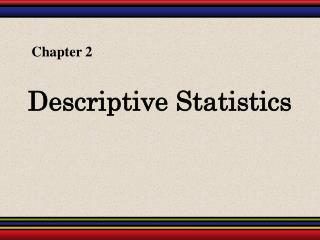DownloadDownload PresentationDescriptive Statistics

# Descriptive Statistics

Télécharger la présentation## Descriptive Statistics

- - - - - - - - - - - - - - - - - - - - - - - - - - - E N D - - - - - - - - - - - - - - - - - - - - - - - - - - -
##### Presentation Transcript

1. Chapter 2 Descriptive Statistics

2. Measures of Position § 2.5

3. Quartiles Median Q1 Q2 Q3 0 25 50 75 100 Q3 is the median of the data above Q2. Q1 is the median of the data below Q2. The three quartiles, Q1, Q2, and Q3, approximately divide an ordered data set into four equal parts.

4. Finding Quartiles Lower half Upper half Q2 Q3 Q1 Example: The quiz scores for 15 students is listed below. Find the first, second and third quartiles of the scores. 28 43 48 51 43 30 55 44 48 33 45 37 37 42 38 Order the data. 28 30 3337 3738 42 43 43 44 45 48 48 51 55 About one fourth of the students scores 37 or less; about one half score 43 or less; and about three fourths score 48 or less.

5. Interquartile Range The interquartile range (IQR) of a data set is the difference between the third and first quartiles. Interquartile range (IQR) = Q3 – Q1. Example: The quartiles for 15 quiz scores are listed below. Find the interquartile range. Q1 = 37 Q2 = 43 Q3 = 48 (IQR) = Q3 – Q1 The quiz scores in the middle portion of the data set vary by at most 11 points. = 48 – 37 = 11

6. Box and Whisker Plot A box-and-whisker plot is an exploratory data analysis tool that highlights the important features of a data set. • The five-number summary is used to draw the graph. • The minimum entry • Q1 • Q2 (median) • Q3 • The maximum entry Example: Use the data from the 15 quiz scores to draw a box-and-whisker plot. 28 30 3337 3738 42 4343 44 45 4848 51 55 Continued.

7. 28 32 36 40 44 48 52 56 Box and Whisker Plot • Five-number summary • The minimum entry • Q1 • Q2 (median) • Q3 • The maximum entry 28 37 43 48 55 Quiz Scores 28 37 43 48 55

8. Percentiles and Deciles Fractiles are numbers that partition, or divide, an ordered data set. Percentiles divide an ordered data set into 100 parts. There are 99 percentiles: P1, P2, P3…P99. Deciles divide an ordered data set into 10 parts. There are 9 deciles: D1, D2, D3…D9. A test score at the 80th percentile (P8), indicates that the test score is greater than 80% of all other test scores and less than or equal to 20% of the scores.

9. Standard Scores The standardscore or z-score, represents the number of standard deviations that a data value, x, falls from the mean, μ. Example: The test scores for all statistics finals at Union College have a mean of 78 and standard deviation of 7. Find the z-score for a.) a test score of 85, b.) a test score of 70, c.) a test score of 78. Continued.

10. Standard Scores Example continued: a.) μ = 78, σ = 7, x = 85 This score is 1 standard deviation higher than the mean. b.) μ = 78, σ = 7, x = 70 This score is 1.14 standard deviations lower than the mean. c.) μ = 78, σ = 7, x = 78 This score is the same as the mean.

11. Relative Z-Scores Example: John received a 75 on a test whose class mean was 73.2 with a standard deviation of 4.5. Samantha received a 68.6 on a test whose class mean was 65 with a standard deviation of 3.9. Which student had the better test score? John’s z-score Samantha’s z-score John’s score was 0.4 standard deviations higher than the mean, while Samantha’s score was 0.92 standard deviations higher than the mean. Samantha’s test score was better than John’s.